# PC221 Analog Electronics I:

## Resistors and Ohmmeter Lab

### Objectives

A resistor is an analog component that is used in most electronic circuits. Before we can put resistors to good use, it is important that you understand how to recognize, identify, and measure a resistor. The objectives for this lab are:
1. To identify fixed resistors and determine their nominal value, tolerance, and expected resistance range.
2. To measure the actual resistance of fixed a resistor using an ohmmeter.
3. To understand the operation of a potentiometer or variable resistor.

### Preparation

Read the section on lab notebooks on this web site. Record all data for this lab in your lab notebook.

Read the section on fixed resistors on this web site. The following charts may also be of use; they all contain the same information but in slightly different formats.

Note: If a resistor has only 3 bands, treat it like a 4 band resistor with no tolerance band. The tolerance for 3 band resistors is 20%.

### Equipment

• digital multimeter - Wavetek 33XR assumed
• 1 4-band colour coded resistors (beige/brown body) of your choice
• 1 5-band colour coded resistors (blue body) of your choice (See 1% resistor bins)
• one of the above resistors should be less than 75 Ω
• trimmer and small screwdriver, any value

### Procedure

#### Resistor Identification

For each of the following sections, record resistor markings and required information in your lab notebook.

1.  The resistors to the right are higher power resistors. Since the resistors are physically large, the nominal value, tolerance, and wattage are written on the resistor. Complete the table.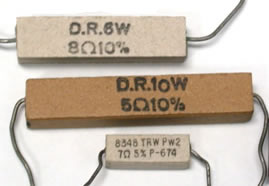nominal value tolerance wattage top resistor 8 Ω ±10% 6 Watts middle resistor bottom resistor 8348 = part number TRW = manufacturer PW= wattage prefix

2.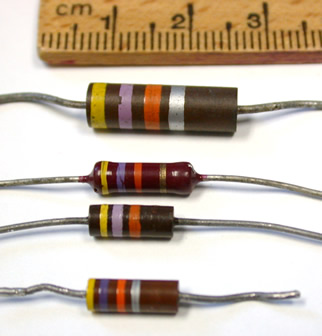[click image to get a larger image] The resistors to the left are carbon composition (3) and carbon film (1). Which one is the carbon film?  How did you determine that? [Note: carbon composition resistors are obsolete.] These resistors use the 4-band colour code to code the resistor's nominal value and tolerance. For the 4-band code, the resistor is oriented so that the right band is always gold, silver, or missing. Complete the table. Of the three carbon composition resistors, which resistor has the highest Wattage? How can you tell? nominal value tolerance expected range top resistor middle top middle bottom bottom resistor 47 kΩ ± 10% 42.3 kΩ - 51.7 kΩ

3. On the bottom resistor, it is difficult to tell if the second band is blue or violet. I have assumed that the band is violet because yellow-blue-orange is not a valid combination for a resistor with a ± 10% tolerance. Why are some colour combinations not valid?

4. #### Resistance Measurement

If you need to know the actual value of the resistor (not the nominal value) or if the colour coding is too difficult to read, it may be necessary to measure the resistance using an ohmmeter, a DMM (Digital MultiMeter) or a VOM (Voltage/Ohm/Milliammeter). The DMM and VOM (analog version of DMM) are multifunction meters that have an ohmmeter function. Read Measuring Resistance for instructions on using the DMM as an ohmmeter before continuing.

Before using an ohmmeter for the first time, determine the meter's symbol for overload. NOTE: depending on the function of the meter, "overload" may or may not be dangerous to the meter. For the ohmmeter function, overload is considered a normal output that indicates an open circuit. An open circuit means that two points are not connected.

To determine how the meter will indicate overload:
• You can look up the symbol in the Operator's Manual. Look it up.
• Alternatively, if you don't have a copy of the manual, use an open circuit to show an overload. Set the meter to ohmmeter (any range) making sure the probes are not touching anything (including each other) and observe the display.

5.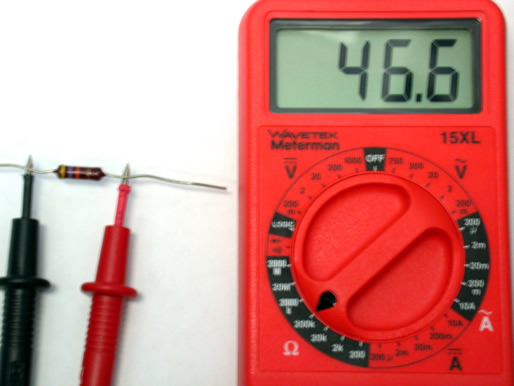For the example shown to the left, the resistor is yellow-violet-orange-gold which corresponds to the nominal value 47,000 ± 5%. The expected resistance range is calculated to be 44.65 kΩ to 49.35 kΩ. A measurement range of 200K is selected because the nominal value of 47 kΩ is between 20KΩ and 200KΩ. Measuring the resistance shows 46.6 on the display with the measurement range at 200K which means the actual resistance is 46.6 kΩ.

For each of the resistors provided for this lab:

• determine the nominal value,
• determine the tolerance,
• calculate the expected resistance range, and
• measure the actual resistance.
Leave space in your table for the true resistance range (next question).

Until you are completely comfortable with the interpretation of the ranges, check the resistor across all of the ohmmeter range values, starting at the lowest range, and record the display at each range value.

Demonstrate resistance measurement to the lab supervisor. Be prepared to explain the associated terminology [e.g. nominal resistance, tolerance, expected resistance range, actual resistance].

Look over the lab so far: Is there a single point of useful information that would be good to include in a lab summary? If so, highlight it.

6. #### Variable Resistors

A potentiometer or variable resistor is like a pair of resistors in series with variable values but a fixed sum. The diagram on the left shows the symbols for a potentiometer. The diagram on the right (hopefully) makes clear the relationship between a potentiometer and the two resistances R1 and R2. (Note: Sometimes a potentiometer is also called a "trimmer".)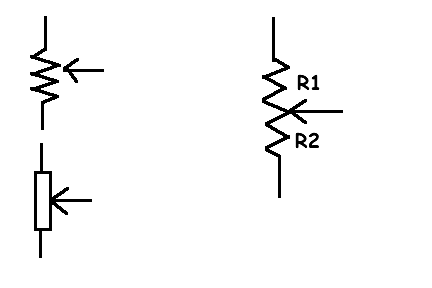The total resistance of the potentiometer, R1 + R2, is constant. The arrow represents a slider or knob which will vary the values of R1 and R2 subject to the constraint that the total resistance does not change. Use an ohmmeter to study the resistance of the (unconnected) potentiometer. Correlate your observations with the diagram above. Determine the minimum and maximum values of R1, R2, and R1+R2.
Do the resistances R1 and R2 vary linearly with the adjustment of the wiper?

7. Note the numbers on the potentiometer. How do they indicate the resistance of the potentiometer? Is it similar to anything else you've seen?

Demonstrate trimmer operation to the lab supervisor. Identify the wiper, and verify that the resistances between the various pins are related as expected.

8. #### Problems with Resistance Measurement

When measuring a very small resistance, it is important to account for the resistance of the meter's leads. Measure the resistance of the leads by touching the two probe tips together.

9. In the instructions for using the digital multimeter, you were cautioned not to include yourself as part of the circuit. [Do not touch the component or the probe tip with your hands as your body's resistance will affect the measurement.] Measure your resistance and that of your partner.
• Make sure you are not grounded (e.g. are not touching the case of any electronic device, are not using a static mat strap) and that you are not part of a circuit.
• Place the meter on the lab bench.
• Stand away from the bench and gently grasp one probe tip in each hand between your thumb and forefinger or gently press the probe tip into the pad of a finger or palm. How you hold the probe will affect the measurement.
• Have your partner select the appropriate scale. It will be very difficult to get a single stable measurement; you will have to take a visual average

How does the measurement change if the contact points on your hands are moist? Resistance will vary from person to person based on the percentage of body fat (fat has a higher resistance) and on their internal hydration (water is a better conductor). This is the principle behind body composition analysis scales and meters.

10. #### Continuity Testing

Use the audible continuity tester mode on the resistors from earlier in the lab. In theory, a resistor should not appear as an electrical connection. What are your results? Why?
A continuity test is actually testing resistance. Therefore, if you do not have a continuity tester, you can use an ohmmeter to test continuity, by looking for a resistance close to zero.

Demonstrate continuity testing to the lab supervisor. Can you determine what threshhold resistance is required in order to not identify as a short circuit?

11. Before you leave the lab, read over the information about lab notebook summaries and the lab summary checklist.
Write out one point for your lab summary, and have the lab instructor verify that it is a good choice. Have the lab instructor sign your lab notebook immediately after your last entry.

## Resources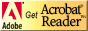If you need to update a browser, you might try Firefox which is
• free
• open source
• available for several platformsSince this page uses cascading style sheets for its layout, it will look best with a browser which supports the specifications as fully as possible.

If you are looking for an office package, with a word processor, spreadsheet, etc., you might try LibreOffice which is
• free
• open source
• available for several platforms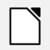Go to the main page for the Department of Physics and Computer Science.

Wilfrid Laurier University